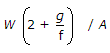# Civil Engineering - Theory of Structures

### Exercise :: Theory of Structures - Section 4

21.

For a strongest rectangular beam cut from a circular log, the ratio of the width and depth, is

 A. 0.303 B. 0.404 C. 0.505 D. 0.606 E. 0.707

Explanation:

No answer description available for this question. Let us discuss.

22.

The moment of inertia of a circular section about any diameter D, is

 A.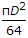B.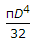C.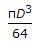D.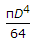Explanation:

No answer description available for this question. Let us discuss.

23.

The ratio of maximum and average shear stresses on a rectangular section, is

 A. 1 B. 1.25 C. 1.5 D. 2 E. 2.5

Explanation:

No answer description available for this question. Let us discuss.

24.

The locus of the moment of inertia about inclined axes to the principal axis, is

 A. straight line B. parabola C. circle D. ellipse.

Explanation:

No answer description available for this question. Let us discuss.

25.

A lift of weight W is lifted by a rope with an acceleration f. If the area of cross-section of the rope is A, the stress in the rope is

 A.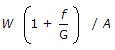B.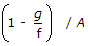C.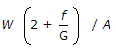D.